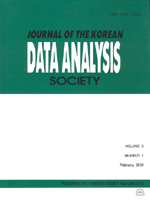상세검색
최근 검색어 전체 삭제
다국어입력
즐겨찾기0KCI등재 학술저널

# Parameter Estimations in a Bernoulli Process

• 등재여부 : KCI등재
• 2005.02
• 73 - 79 (7 pages)

In this paper, we study the parameter estimation in Bernoulli distribution and Geometric distribution with parameter . We consider the estimator of and we find two confidence intervals for the parameters in these distributions. One is the result of variance stabilization(V. S.) and the other is that of using central limit theorem(C. L. T.). Simulation results shows that the point estimates are not so different and the length of the confidence intervals obtained by V. S. in case of geometric distribution is smaller than that by C. L. T.

1. Introduction

2. Main Results

3. Simulation Results

References